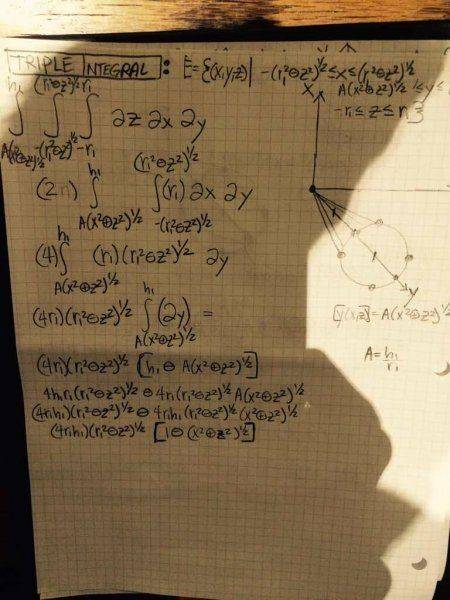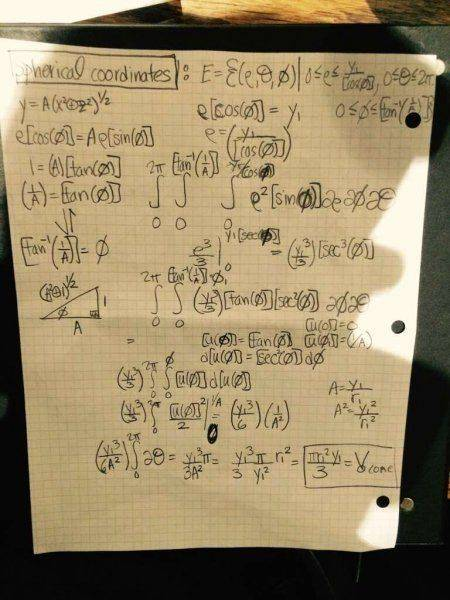# Double integral in Rectangular coordinates for anything circular

• PaultheRiemann

#### PaultheRiemann

This is the equation for the cone
A \sqrt{x^2 + y^2}

The double integral

\iint A \sqrt{x^2 + y^2} \space dy \space dx \space \space \space

\text {From x= -1 to 1 and y=} -\sqrt{1-x^2} \space to \space \sqrt{1-x^2}

\text{ is very difficult to evaluate. I've tried polar coordinate substitution. However, I can't seem to get} /space 2/3 \pi z \space \text{where z is the height of cone and 1 is the radius of the cone}

\text{here's what happens when I use CLAIRAUT theorem to change the order of the pertaining triple integral}I'm also trying my best not to use cylindrical and spherical coordinate systems.easy when spherical coordinates are used

You are not getting any responses, perhaps because no one can understand what you are saying.
Put your Latex between double dollar signs.
This is the equation for the cone
$$A \sqrt{x^2 + y^2}$$
No, it isn't, in fact, it is not even an equation! I assume you meant $$z= A\sqrt{x^2+ y^2}$$ but even that is only one side of the cone- the entire cone is $$z^2= A^2(x^2+ y^2)$$

The double integral

$$\iint A \sqrt{x^2 + y^2} \space dy \space dx \space \space \space$$

$$\text {From x= -1 to 1 and y=} -\sqrt{1-x^2} \space to \space \sqrt{1-x^2}$$

\text{ is very difficult to evaluate. I've tried polar coordinate substitution. However, I can't seem to get} /space 2/3 \pi z \space \text{where z is the height of cone and 1 is the radius of the cone}
To integrate $\int_{x= -1}^1\int_{y= {-\sqrt{1- x^2}}^\sqrt{1- x^2} A\sqrt{x^2+ y^2}dy dx$$I would first use trig identity$$tan^2(\theta)+ 1= sec^2(\theta)$$and make the substitution$$y= xtan(\theta)$$. Then$$\sqrt{x^2+ y^2}= \sqrt{x^2+ x^2 tan(\theta)}= x sec(\theta)$$. Also$$dy= sec^2(\theta)d\theta$$This is the equation for the cone A \sqrt{x^2 + y^2} The double integral \iint A \sqrt{x^2 + y^2} \space dy \space dx \space \space \space \text {From x= -1 to 1 and y=} -\sqrt{1-x^2} \space to \space \sqrt{1-x^2} \text{ is very difficult to evaluate. I've tried polar coordinate substitution. However, I can't seem to get} /space 2/3 \pi z \space \text{where z is the height of cone and 1 is the radius of the cone} No, it is not that difficult. The substitution$$y= x tan(\theta)$$works nicely. But I don't see why you are doing that. if you want to find the volume of a cone, with height h, then the double integral you are using isn't going to do it. Instead, note that, for any z, a cross section is a disk with center (0, 0, z) and radius$$r= \sqrt{x^2+ y^2}= \frac{z}{A}$$so area$$\pi \frac{z^2}{A^2}$$. Taking the "thickness" to be dz, the volume of such disk is$$\pi \frac{z^2}{A^2}dz$$. The entire cone, thought of as the sum of such disks is$$\frac{\pi}{A^2}\int_0^h z^2 dz$$. In this particular cone, with$$z= A\sqrt{x^2+ y^2}$$a cone with height h will have base radius$$\frac{h}{A}$$. That will NOT give "$$\frac{2}{3}\pi h$$" because that is NOT the formula for volume of a cone. The volume of a cone, of height h and base radius R, is$$\frac{1}{3}\pi r^2h$\$- 1/3, not 2/3.

Thanks halls